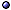Quantum Geometrodynamics: Dynamics in Superspace

The Program in General > s.a. canonical general relativity and ADM formulation [classical version]; canonical quantum gravity.
* Idea: Geometrodynamics is the program for canonical quantum gravity based on superspace and the dynamics given there by the Wheeler-DeWitt equation; A 4-geometry is represented by a classical set of points C of superspace, contained in it as a hypersurface; The set of 3D geometries contains the time information; Initial data consists of a neighborhood in C, from which the Einstein equation gives C.
* Problems: Mainly interpretational, such as What is the meaning of Ψ(q, φ)? We need an inner product for Ψs before we say that |Ψ|2 is a probability.

Kinematics: Superspace > s.a. canonical quantum gravity; regge calculus [simplicial superspace]; topology change.
* Idea: Superspace is a reduced configuration space for a Hamiltonian formulation of gravity, in which a point is a diffeomorphism equivalence class of Riemannian metrics on a spacelike hypersurface Σ: S(Σ):= Riem(Σ)/Diff(Σ).
* Constraints: This choice of configuration space eliminates the diffeomorphism constraint, but the scalar one (coming from the gauge arbitrariness in choosing a slicing of spacetime) remains, and becomes the dynamical equation.
* Topology: S(Σ) is not a manifold, but is made up out of a finite number of manifolds (strata).
* Extended: Glue together copies of the same space [@ DeWitt (70)].
* Grand: Need singular geometries.
* Minisuperspace: Impose enough symmetries to get finite number of degrees of freedom.
@ Structure of superspace: & Peres < 79; Fischer in(70); in Brill in(72); Reula pr(GR12); Christodoulakis & Zanelli pr(89); Giulini HPA(95)gq/93, PRD(95)gq/93; Fischer & Moncrief GRG(96) [including conformal superspace]; Giulini GRG(09)-a0902; Gomes JMP(11); Liu a1509 [Gromov's ε-approximation topology].
@ Supermetric: Pekonen JGP(87); Szydłowski JMP(99); Schmidt gq/01-proc; Hehl & Kiefer GRG(18)-a1711 [comparison with the 4th-rank constitutive tensors in electrodynamics and elasticity theory].
@ Minisuperspace: Misner in(72); Kuchař & Ryan in(86); Kiefer AP(91); Kerbrat et al RPMP(92); Saremi gq/01; > s.a. gowdy spacetimes.
@ Related topics: Jacobson in(88) [self-dual representation]; Rainer gq/96-conf [locally homogeneous]; Barbour & Ó Murchadha a1009 [conformal superspace]; > s.a. Configuration Space; riemannian geometry.

States: Wave Functionals > s.a. time in quantum gravity.
* Idea: States are functionals Ψ[qab,φ] of metrics (and matter fields) belonging to an appropriate space; Notice that these states will end up without a time dependence, since they must satisfy the constraints; Instead, time can be taken as one of the components of qab.
@ Inner product: DeWitt PR(67); Tsamis & Woodard PRD(87); Christodoulakis & Zanelli CQG(87); Vilenkin PRD(89).

Dynamics: Wheeler-DeWitt Equation > s.a. 3D quantum gravity; cosmological constant; FLRW quantum cosmology; lattice gravity [discretized].
* Idea: The "zero-energy" Schrödinger-like equation one gets as the operator version of the scalar constraint, by replacing q and p by their coordinate representation operators (multiplication operator and −i$$\hbar$$(δ/δq), respectively), and acting with it on a wave functional Ψ[qab, φ].
* Expression: For the dynamics of gravity coupled to a field φ, it is

{−Gabcd2qab δqcd) + q1/2[–3R + 2Λ + (16πG)−1 T00(iδ/δφ, φ)]} Ψ(q, φ) = 0 ,

up to linear derivative terms depending on the choice of factor ordering, where the supermetric, with "signature" (−, +, +, +, +, +) –the minus sign coming from the conformal mode– and thought of as a 6 × 6 matrix, is defined by

Gabcd = $$1\over2$$q−1/2 (qac qbd + qad qbcqab qcd) .

@ General references: Woodard CQG(93); Jackiw gq/95 [modified]; Błaut & Kowalski-Glikman gq/96 [solutions, and quantum potential]; Norbury EJP(98)phy [II, from Newtonian physics]; Soo CQG(07)gq [in terms of gauge-invariant 3-geometry elements]; Sawayama a0904 [small universe]; Cherkas & Kalashnikov DANB-a1406 [near small scale factors, discrete vs continuum spectrum]; Rovelli CQG(15)-a1506 [rev].
@ Related topics: Gurzadyan & Kocharyan JETP(87), Kocharyan CMP(91) [stability of cosmological models]; Dereli et al PLB(94) [as spinor equation, including degenerate metrics and signature change]; Mehta a1912 [in null-foliated spacetimes].

References > s.a. quantum cosmology [measure, third quantization]; semiclassical quantum gravity; supergravity; supersymmetric field theories.
@ General: Wheeler 62; Baierlein et al PR(62); Fletcher in(62); Marzke & Wheeler in(64); Wheeler in(64); DeWitt PR(67); Wheeler in(68); Wheeler 68; Brill & Gowdy RPP(70); DeWitt in(70); Fischer in(70); Misner in(72); Christodoulou & Francaviglia AAST(76); Shestakova a0801-proc, MG12(12)-a0911 [problems and approaches to their solution]; Kiefer GRG(09)-a0812 [overview]; Shestakova G&C(19)-a1912 [and gauge invariance].
@ Operator ordering: Hawking & Page NPB(86); Kontoleon & Wiltshire PRD(99)gq/98; Hall GRG(05)gq/04-in [from "exact uncertainty"]; Huang et al EPJC(16)-a1505 [and the existence of a classical universe].
@ And time evolution: Kheyfets & Miller gq/94, IJMPA(00); Cosgrove CQG(96)gq/95 [time slicings and consistency]; Gil-Medrano JMP(96); Gentle et al IJMPA(04)gq/03, George et al gq/03-proc [constraints and true degrees of freedom]; Ita et al PTEP(15)-a1501 [with intrinsic time].
@ Relationships: Kubota et al PLB(04) [Wheeler-DeWitt and AdS-cft]; Gielen Sigma(11)-a1111 [and connection dynamics].
@ WKB approximation: Gerlach PR(69); Horiguchi NCB(96).
@ Related topics: Parentani NPB(97)gq/96 [and Green functions for matter fields]; Carroll gq/05 [uncertainties and statistical geometrodynamics]; Nelson & Sakellariadou PLB(08)-a0709 [quantum corrections and effective matter]; Pedram & Jalalzadeh PRD(08) [signature change with fluids]].
@ Generalizations: Shestakova G&C(99)gq/00, G&C(05) [extended phase space], G&C(00)gq [and cosmological constant], in(10)-a0810; Belinchón IJMPD(02)gq/01 [varying constants]; Brännlund et al CQG(14)-a1312 [topology and volume effects]; Faizal IJMPA(14)-a1406, IJMPA(15)-a1503 [deformed canonical commutation relations]; Anderson a1412 [space of spaces].Based on modified gravity: see phenomenology [with non-linear correction]; lattice gravity and regge calculus [discrete versions]; modified approaches [scalar-tensor].